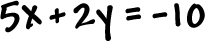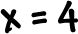Here's what we've learned so far about graphing lines: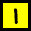If it's in the formgraph it by finding the intercepts.If it's in the form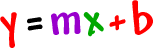start at y = b and, then, use the slope (rise over run) to nail the second point.If it's in the formthen it's a horizontal line.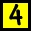If it's in the formthen it's a vertical line.

Do all the problems below, then there's one glitch I need to tell you about.

TRY IT:

Graph